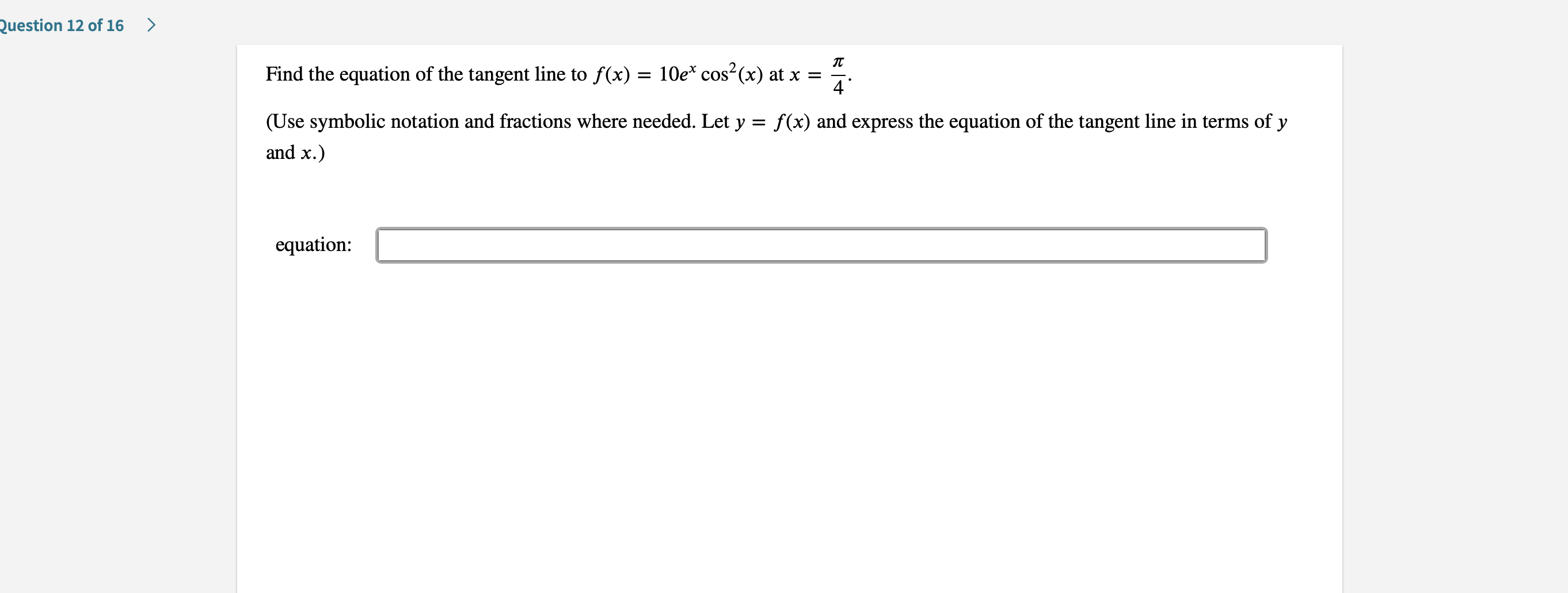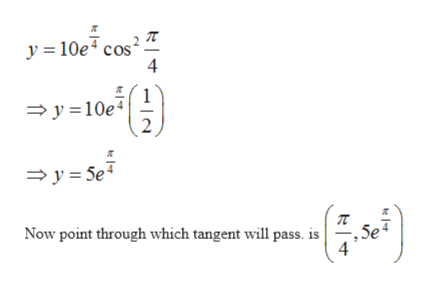# Question 12 of 16>Find the equation of the tangent line to f(x) = 10e* cos2(x) at x4(Use symbolic notation and fractions where needed. Let y f(x) and express the equation of the tangent line in terms of y=and x.)equation

Question
6 viewshelp_outlineImage TranscriptioncloseQuestion 12 of 16 > Find the equation of the tangent line to f(x) = 10e* cos2(x) at x 4 (Use symbolic notation and fractions where needed. Let y f(x) and express the equation of the tangent line in terms of y = and x.) equation fullscreen
check_circle

Step 1

To find equation of the tangent to the given function at a given point

Given function is

Step 2

And x coordinate of the point is given as

Step 3

First find out the ...help_outlineImage Transcriptionclosey 10e cos 4 1 y10e 2 y 5e - Now point through which tangent will pass. is , 5e 4 fullscreen

### Want to see the full answer?

See Solution

#### Want to see this answer and more?

Solutions are written by subject experts who are available 24/7. Questions are typically answered within 1 hour.*

See Solution
*Response times may vary by subject and question.
Tagged in

### Other